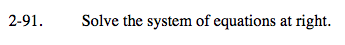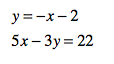Home > A2C > Chapter 2 > Lesson 2.1.6 > Problem2-91

2-91.
1. Solve the system of equations below. Homework Help ✎

2. y = −x − 2
5x − 3y = 22Substitute the first equation for y in the second equation.

5x − 3(−x − 2) = 22
5x + 3x + 6 = 22
8x + 6 = 22
8x + 6 − 6 = 22 − 6
8x = 16
x = 2
Now substitute 2 into the first equation for y and evaluate for x.